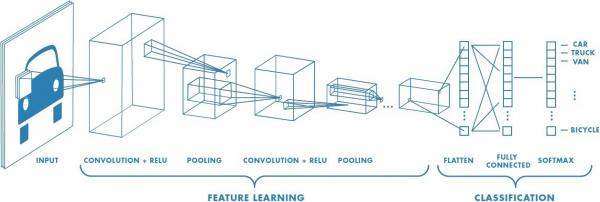Yashb | What is Convolutional Neural Networks (CNNs)?

# What is Convolutional Neural Networks (CNNs)?

Mark B 13 Feb, 2023 3 minConvolutional Neural Networks (CNNs) are a type of deep learning neural network architecture that are widely used for image and video recognition tasks. They are designed to process data with a grid-like structure, such as an image, and learn features from the data that are relevant for the task at hand.

CNNs consist of several layers, including the input layer, hidden layers, and output layer. The input layer is where the data is fed into the network. The hidden layers are where the convolutional, activation and pooling operations take place. The output layer is where the network produces its predictions.

The convolutional layer is the main building block of a CNN. In this layer, the network applies filters to the input data, producing feature maps that capture specific patterns in the data. These feature maps are then processed by activation functions, which introduce non-linearity into the network and allow it to learn complex relationships in the data.

The pooling layer is another important component of a CNN. It reduces the size of the feature maps, which helps to reduce the number of parameters in the network and improve its computational efficiency. The pooling layer also helps to reduce overfitting, which is a common problem in deep learning.

One of the key benefits of CNNs is their ability to learn hierarchical representations of data. This means that they can learn high-level features, such as the shape of an object, and lower-level features, such as its texture, by processing the data through multiple layers. This allows them to perform well on a wide range of image and video recognition tasks, such as object detection, image classification, and semantic segmentation.

In recent years, CNNs have been used to achieve state-of-the-art performance on many image and video recognition benchmarks. They have also been applied to other domains, such as speech recognition and natural language processing, where they have achieved similarly impressive results.

## Real Life Uses

Image Classification: CNNs are widely used for image classification tasks, such as recognizing objects in images, identifying faces, and classifying images into different categories.

Object Detection: CNNs can also be used for object detection in images and videos, such as detecting cars, pedestrians, and other objects in self-driving cars.

Medical Imaging: In medical imaging, CNNs are used for tasks such as segmentation, diagnosis, and classification of diseases and conditions. For example, they can be used to identify tumors in magnetic resonance imaging (MRI) scans or classify skin lesions as benign or malignant.

Natural Language Processing: CNNs have also been used in Natural Language Processing (NLP) tasks, such as sentiment analysis, text classification, and language translation.

Computer Vision: CNNs are used in various computer vision applications, such as video analysis, image super-resolution, and optical character recognition (OCR). For example, they can be used to track objects in videos or enhance the resolution of low-quality images.

## Python Example

``````import numpy as np

import keras

from keras.datasets import mnist

from keras.models import Sequential

from keras.layers import Dense, Dropout, Flatten

from keras.layers import Conv2D, MaxPooling2D

from keras import backend as K

(x_train, y_train), (x_test, y_test) = mnist.load_data()

# Preprocess the data

# Reshape the data to (number of samples, 28, 28, 1)

x_train = x_train.reshape(x_train.shape, 28, 28, 1)

x_test = x_test.reshape(x_test.shape, 28, 28, 1)

# Convert data type to float32

x_train = x_train.astype('float32')

x_test = x_test.astype('float32')

# Normalize the data to [0, 1]

x_train /= 255

x_test /= 255

# Convert the labels to categorical

y_train = keras.utils.to_categorical(y_train, 10)

y_test = keras.utils.to_categorical(y_test, 10)

# Define the model

model = Sequential()

# Add a Conv2D layer with 32 filters of size (3, 3) and ReLU
activation

input_shape=(28, 28, 1)))

# Add a MaxPooling2D layer with pool size (2, 2)

# Flatten the output of the previous layer to 1D

# Add a dense layer with 128 units and ReLU activation

# Add a dropout layer with rate 0.5 to prevent overfitting

# Add the output layer with 10 units and softmax activation

# Compile the model

# Use categorical crossentropy as the loss function

# Use accuracy as the metric

model.compile(loss='categorical_crossentropy',

# Train the model

# Train for 10 epochs with batch size 32

model.fit(x_train, y_train, batch_size=32, epochs=10,
verbose=1, validation_data=(x_test, y_test))

# Evaluate the model on the test data

score = model.evaluate(x_test, y_test, verbose=0)

print('Test loss:', score)

print('Test accuracy:', score)
``````

This code demonstrates how to define and train a simple CNN using the MNIST dataset, which contains handwritten digits. The model consists of a series of convolutional and pooling layers, followed by fully connected layers, and uses the ReLU activation function and categorical cross entropy loss. The model is trained using the Adam optimizer and evaluated on the test data to measure its accuracy.

Note that this is just a simple example code and there are many ways to customize and improve the model, such as changing the architecture, using different activation functions, and using different loss functions and optimizers. The goal of this code is to provide a starting point for those interested in exploring CNNs and the Keras library.

To conclude, Convolutional Neural Networks (CNNs) are a powerful and widely used type of deep learning neural network architecture that have shown impressive results on a wide range of image and video recognition tasks. They are able to learn hierarchical representations of data, which enables them to perform well on these tasks, and are an important tool for researchers and practitioners in the field of artificial intelligence.